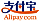Bookcover of Differential Geometry and Electromagnetism
Booktitle:

# Differential Geometry and Electromagnetism

LAP LAMBERT Academic Publishing (2013-09-19 )eligible for voucher
ISBN-13:

### 978-3-659-47352-4

ISBN-10:
3659473529
EAN:
9783659473524
Book language:
English
Blurb/Shorttext:
These lecture notes correspond to a short course on Mathematics focusing on the differential geometry of compact manifolds, the exterior Clifford algebra of differential forms and their applications to the classical and relativist theories of electromagnetism in Physics. It is assumed that the reader already knows the definitions and techniques of the exterior calculus and the integration theorems of differential forms on manifolds and submanifolds of euclidean spaces. The text is divided in four chapters. Except Chapter 1, which is an introduction, each chapter is independent from the others. Chapter 2 studies the integration theorems that are obtained from the differential statements of classical Maxwell's equations. In Chapter 3, the Lorentz transformations are introduced to prove the relativist theorems of electromagnetism. Finally, in Section 4, Poisson's equation is introduced and solved by global integration.
Publishing house:
Website:
https://www.lap-publishing.com/
By (author) :
Eleonora Catsigeras
Number of pages:
64
Published at:
2013-09-19
Stock:
Available
Category:
Mathematics
Price:
23.90 €
Keywords:
Differential Forms, Electromagnetic Theory, exterior calculus### Categories